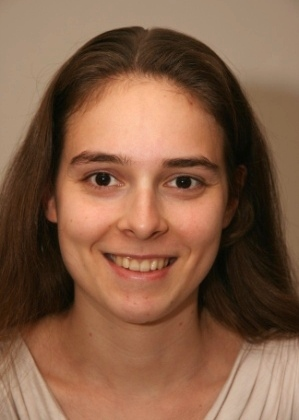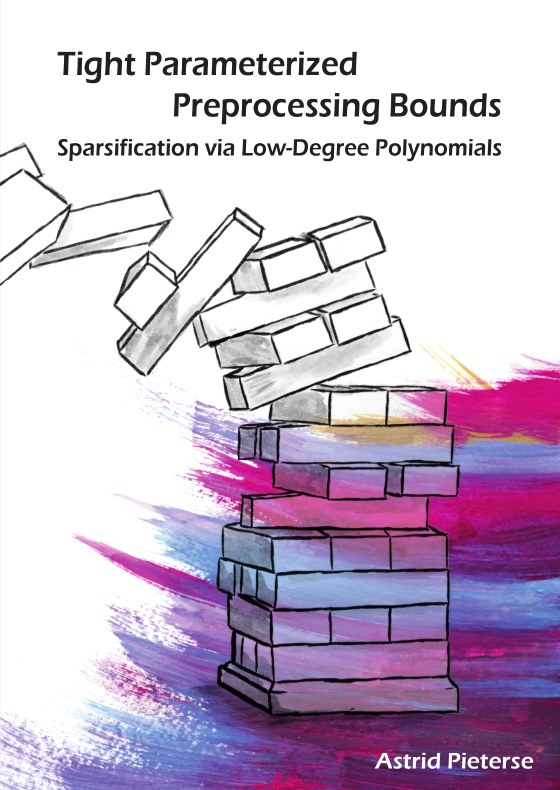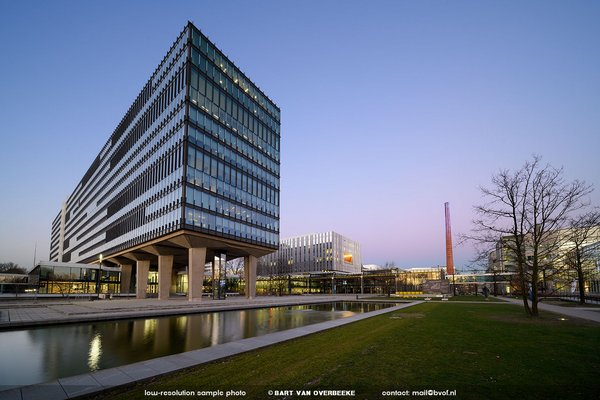# PhD Defense Astrid Pieterse

On September 4th Astrid Pieterse will defend her PhD thesis entitled 'Tight Parameterized Preprocessing Bounds: Sparsification via Low-Degree Polynomials'.Astrid's promotors are Mark de Berg and Bart Jansen.

The defense will take place at 16:00 hrs in room Atlas 0.710 of the Eindhoven University of Technology.

## AbstractMany of the problems we are interested in solving algorithmically are known to be NP-hard and can (probably) not be solved efficiently. In this thesis we will study preprocessing algorithms for such problems. In particular, we will be interested in polynomial-time preprocessing algorithms that guarantee that the preprocessed instance is equivalent to the original one, and that its size is bounded by a function of some parameter of the original instance. Such preprocessing algorithms are known as kernels, and the bound on the size of the resulting instance is the size of the kernel. Naturally, one can ask what the best-possible size is for a certain problem, with a certain parameter. In this thesis, we study two types of problems.

First of all, we study the very general constraint satisfaction problem. Given a number of constraints over a set of variables, the problem asks to set values to the variables that satisfy all constraints. We obtain kernel upper and lower bounds, depending on the type of constraints that occur in an instance.

Secondly, we give kernel upper and lower bounds for a graph coloring problem, where the question is to color the vertices in a graph such that this coloring satisfies certain requirements. For example, we may require that adjacent vertices receive distinct colors.

You can read the thesis here.

Details
When: Wednesday September 4th, 2019
Time: 16:00
Where: TU/e
LocationEindhoven University of

Technology
Atlas building, room 0.710